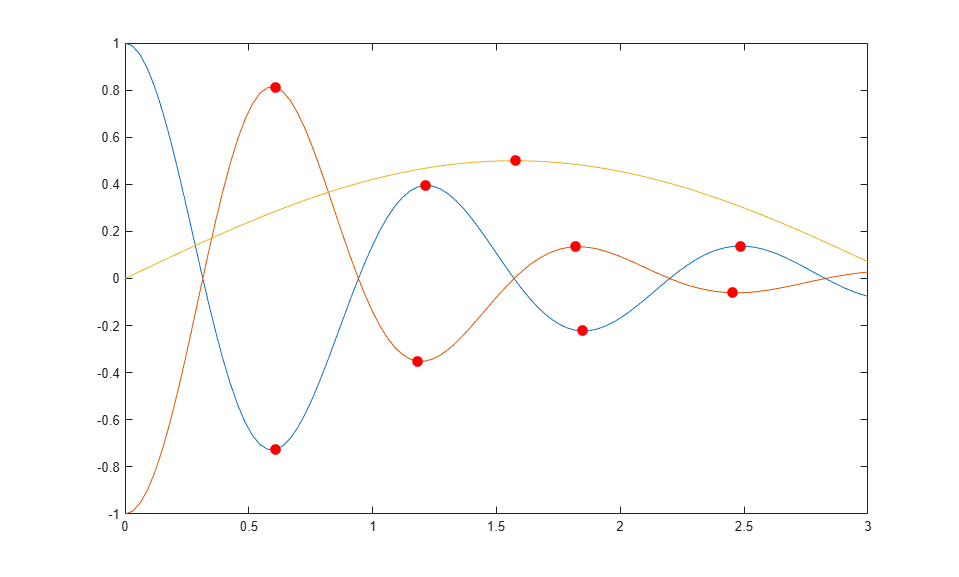# Chart Class with Variable Number of Lines

This example shows how to define a class of charts that can display any number of lines based on the size of the user's data. The chart displays as many lines as there are columns in the `YData` matrix. For each line, the chart calculates the local extrema and indicates their locations with circular markers. The following code demonstrates how to:

• Define two properties called `PlotLineArray` and `ExtremaArray` that store the objects for the lines and the markers, respectively.

• Implement an `update` method that replaces the contents of the `PlotLineArray` and `ExtremaArray` properties with the new objects. Because this method executes all the plotting and configuration commands, the `setup` method is empty. This is a simple way to create any number of lines. To learn how to create this chart more efficiently, by reusing existing line objects, see Optimized Chart Class for Displaying Variable Number of Lines.

To define the class, copy this code into the editor and save it with the name `LocalExtremaChart.m` in a writable folder.

```classdef LocalExtremaChart < matlab.graphics.chartcontainer.ChartContainer % c = LocalExtremaChart('XData',X,'YData',Y,Name,Value,...) % plots one line with markers at local extrema for every column of matrix Y. % You can also specify the additonal name-value arguments, 'MarkerColor' % and 'MarkerSize'. properties XData (1,:) double = NaN YData (:,:) double = NaN MarkerColor {validatecolor} = [1 0 0] MarkerSize (1,1) double = 5 end properties(Access = private,Transient,NonCopyable) PlotLineArray (:,1) matlab.graphics.chart.primitive.Line ExtremaArray (:,1) matlab.graphics.chart.primitive.Line end methods(Access = protected) function setup(~) end function update(obj) % get the axes ax = getAxes(obj); % Plot Lines and the local extrema obj.PlotLineArray = plot(ax,obj.XData,obj.YData); hold(ax,'on') % Replicate x-coordinate vectors to match size of YData newx = repmat(obj.XData(:),1,size(obj.YData,2)); % Find local minima and maxima and plot markers tfmin = islocalmin(obj.YData,1); tfmax = islocalmax(obj.YData,1); obj.ExtremaArray = plot(ax,newx(tfmin),obj.YData(tfmin),'o',... newx(tfmax),obj.YData(tfmax),'o',... 'MarkerEdgeColor','none',... 'MarkerFaceColor',obj.MarkerColor,... 'MarkerSize',obj.MarkerSize); hold(ax,'off') end end end ```

After saving the class file, you can create an instance of the chart. For example:

```x = linspace(0,3); y1 = cos(5*x)./(1+x.^2); y2 = -cos(5*x)./(1+x.^3); y3 = sin(x)./2; y = [y1' y2' y3']; c = LocalExtremaChart('XData',x,'YData',y); ```Change the marker size to `8`.

```c.MarkerSize = 8; ```